## Bobbing Melody

So finally, I present to you my magical, whimsical, and bobbing art piece that will let you make your own melody.  So here’s the Bobbing melody in action.

To explain the implementation, here is the Arduino circuit.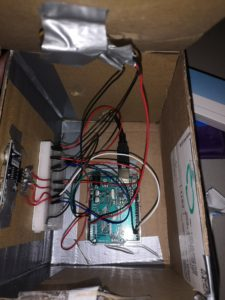The potentiometer values are mapped to create a color wheel (the red knob) that will control the color of visualization in real-time. The values of the ultrasonic sensor fitted in the mysterious black box are mapped into the x-axis for visualization. So the hand position in front of the screen is the position of the new balls dropping from the top of the screen. In visualization, these balls bounce at the bottom of the screen and upon hitting the bottom, the color of the just hit ball changes to yellow to eventually later fade back into its original color.  The whole screen is divided into twenty-one different notes of a piano and the x position of the hit ball determines the corresponding note to play. The music for piano is implemented using MIDI library and using tools like sforzando and loopMIDI.

While this project was very challenging and frustrating at times to finish, I surely had a great time working on it.  I am really glad how it turned out. A lot of the parts, especially the visualization was a trial and error process. I played around with a lot of color schemes and the speed and frame rate, took a lot of time, but finalized on my current settings and I love it. I hope you enjoyed this too.  If time permits, I would love to work on this project more to have various different instruments and visualizations to create a whole tune using different musical instruments and different visualization. Surely, this project and the class have inspired me a lot creatively.

Below is the code for Processing and Arduino.

```//PROCESSING
//Serial connection and sound
import processing.serial.*;
import themidibus.*;
MidiBus myBus;
Serial myPort;

//variables for color mapping
int count = 400;
float radToDeg = 180.0 / PI;
float countToRad = TWO_PI / float(count);
float currentcolor;
float Xslider;

//array list for balls
ArrayList<Ball> balls;

//sounds 21
int[] pitches = {48,52,53,55,59,60,64,65,67,71,72,76,77,79,83,84,88,89,91,95,96};

void setup() {
fullScreen();
//size(800,600);
noStroke();
frameRate(54);

//port communication
String portname=Serial.list();
myPort = new Serial(this,portname,9600);

//midibus
myBus = new MidiBus(this,-1,"loopMIDI Port");

// Create an empty ArrayList (will store Ball objects)
balls = new ArrayList<Ball>();

//color mode
colorMode(HSB,360,99,99);

}

void draw() {
background(0,0,0);

}

//display all balls in action
for (int i = balls.size()-1; i >= 0; i--) {
Ball ball = balls.get(i);
if(ball.colorhue != 0){
ball.move();
}
if(ball.colorhue != 0){
ball.display();
}

//if no motion or very away from slider, reduce their life
if(ball.x <= Xslider-width/6|| ball.x >= Xslider+width/6 || fade==true){
if(ball.life > 100){
ball.life = 100;
}
}

//sound and colorshift on bouncing back from bottom
if(ball.y >= height){
ball.colorhue = 60;
int numsound = int(map(ball.x,0,width,0,19));
if(numsound<0 || numsound>20){
numsound = abs(numsound%21);
}
if(ball.life<100){
myBus.sendNoteOn(0,pitches[numsound],50);
}
else{
myBus.sendNoteOn(0,pitches[numsound],127);
}
}

//set the color back to orignal
if(ball.y < height-height/9){
ball.colorhue = ball.originalhue;
}

//remove the balls from array with finished lives
if (ball.finished()) {
balls.remove(i);
}
}
}

// A new set of x ball objects are added to the ArrayList
for(int i=0; i<count; i++){
float randomS = random(0,10);
float ballWidth = random(4,30);
float xPos = random(Xslider-width/20, Xslider+width/20);
float yPos = random(-20,0);
float angle = currentcolor * countToRad;
int hue = int(angle * radToDeg);
}
}

//Serial Communication
void serialEvent(Serial myPort){
s=trim(s);
if (s!=null){

int values[]=int(split(s,','));
if (values.length==2){
currentcolor = map(values,19,925,380,290);
print(values);
print(",");
println(values);
if(values>150 && values<3000){
Xslider= map(values,150,3000,width, 0);
}
else{
}
}
}
}

// Simple bouncing ball class

class Ball {

float x;
float y;
float speed;
float acceleration;
float w;
float life = 255;
int colorhue;
int originalhue;
float drag;
boolean bounced= false;

Ball(float tempX, float tempY, float tempW, float tempS, int hue) {
x = tempX;
y = tempY;
w = tempW;
speed = tempS;
colorhue = hue;
originalhue = hue;
acceleration = 0.3;
drag = 0.99;
}

void move() {
speed = speed + acceleration;
speed = speed * drag;

// Add speed to y location
y = y + speed;

// If ball reaches the bottom
// Reverse speed
if (y > height) {
// Dampening
speed = speed * -0.9;
y = height;
bounced = true;
}

if(bounced==true && y<=10){
// Dampening
speed = speed * -0.9;
y = 10;
bounced = false;
}
}

boolean finished() {
life--;
if (life < 0) {
return true;
} else {
return false;
}
}

void display() {
// Display the circle
if(colorhue == 0){
fill(color(colorhue,0,99),life);
}
else{
fill(color(colorhue,99,99),life);
}
ellipse(x,y,w,w);
}
}

```
```//ARDUINO

int trigger_pin = 2;
int echo_pin = 3;
long distance, pulse_duration;
int starttime;

void setup() {
Serial.begin(9600);
pinMode(trigger_pin, OUTPUT);
pinMode(echo_pin, INPUT);
digitalWrite(trigger_pin, LOW);
Serial.println("0,0");
}

void loop() {
digitalWrite(trigger_pin, HIGH);
digitalWrite(trigger_pin, LOW);
pulse_duration = pulseIn(echo_pin, HIGH);
Serial.print(sensor);
Serial.print(',');
Serial.println(pulse_duration);
}```

## Final Project 90% Status Update

Idea

As you have read previously, I planned on creating a generative interactive art piece. However, with frequent feedback and suggestions, the idea is now more of a piano with art on screen.  The distance sensor on Arduino helps to identify from where to drop balls from the top and bounce on screen and the potentiometer allows the user to select the color of the balls. As soon as the user moves hands away from the setup, the art form stops with the new production of balls. The sound to be played is dependent on where exactly on the bottom the balls hit.

To stick with a theme, the color palette is defined to be following:One concern is that the colors on the screen are different from what was printed on the knob. I’m not sure how I can fix this but I hope it is not a big issue.

The tunes were collected as samples but still require some more work to have a better general music sound. The overall interaction design (that includes a bigger circuit wiring and piano theme cardboard layout) is still under process.

User Testing

Feedback

• add different shapes (will try and depending on better aesthetic will finalize)
• allow for faster response
• better positioning of distance sensor
• fun to play

Code

```//Main Project Processing File
//Serial connection and sound
import processing.serial.*;
import processing.sound.*;
Serial myPort;

//variables for color mapping
int count = 400;
float radToDeg = 180.0 / PI;
float countToRad = TWO_PI / float(count);
float currentcolor;
float Xslider;

boolean playing = false;

//array list for balls
ArrayList<Ball> balls;

//sounds
SoundFile[] files;
int numsounds = 10;

void setup() {
fullScreen();
//size(800,600);
noStroke();
frameRate(25);

//port communication
String portname=Serial.list();
myPort = new Serial(this,portname,9600);

// Create an empty ArrayList (will store Ball objects)
balls = new ArrayList<Ball>();

//sounds
files = new SoundFile[numsounds];
for (int i = 0; i < numsounds; i++) {
files[i] = new SoundFile(this, (i) + ".wav");
};
colorMode(HSB,360,99,99);

}

void draw() {
background(0,0,99);
}

for (int i = balls.size()-1; i >= 0; i--) {
Ball ball = balls.get(i);
ball.move();
ball.display();
if(ball.x <= Xslider-width/5 || ball.x >= Xslider+width/5 || fade==true){
if(ball.life > 200){
ball.life = 150;

}
}
if(ball.y >= height){
int numsound = int(map(ball.x,0,width,0,9));
playSounds(numsound);
}
if (ball.finished()) {
balls.remove(i);
}
}
}

void playSounds(int numsound){
if(numsound>=0 && numsound<=9 && !files[numsound].isPlaying() ){
files[numsound].play();
}
for(int i=0;i<10;i++){
if(files[i].isPlaying() && i!=numsound){
if(frameCount%20==0){
files[i].stop();
}
}
}
}

// A new set of x ball objects are added to the ArrayList
for(int i=0; i<count; i++){
float randomS = random(3,10);
float ballWidth = random(4,30);
float xPos = random(Xslider-width/10, Xslider+width/10);
float yPos = random(-100,0);
float angle = currentcolor * countToRad;
int hue = int(angle * radToDeg);
}
}

//Serial Communication
void serialEvent(Serial myPort){
s=trim(s);
if (s!=null){

int values[]=int(split(s,','));
if (values.length==2){
currentcolor = map(values,0,1023,290,380);
if(values>150 && values<1700){
Xslider= map(values,150,1700,width, 0);
}
else{
}
}
}
}```
```// Simple bouncing ball class

float noiseScale = 700;

class Ball {

float x;
float y;
float speed;
float gravity;
float w;
float life = 370;
int colorhue;
boolean bounced= false;

Ball(float tempX, float tempY, float tempW, float tempS, int hue) {
x = tempX;
y = tempY;
w = tempW;
speed = tempS;
colorhue = hue;
gravity = 0.1;
}

void move() {
speed = speed + gravity;
// Add speed to y location
y = y + speed;
float noiseVal = noise(x*noiseScale);
int sign;
if(Xslider<width/2){
sign = -1;
}
else{
sign = 1;
}
noiseVal = sign * noiseVal;

// If ball reaches the bottom
// Reverse speed
if (y > height) {
// Dampening
speed = speed * -0.9;
y = height;
bounced = true;
}

if(bounced==true){
x = (noiseVal+x);
}
if(bounced==true && y<=10){
// Dampening
speed = speed * -0.9;
y = 10;
bounced = false;
}
}

boolean finished() {
life--;
if (life < 0) {
return true;
} else {
return false;
}
}

void display() {
// Display the circle
fill(color(colorhue,99,99),life);
ellipse(x,y,w,w);
}
}```
```//Arduino Code
int trigger_pin = 2;
int echo_pin = 3;
long pulse_duration;

void setup() {
Serial.begin(9600);
pinMode(trigger_pin, OUTPUT);
pinMode(echo_pin, INPUT);
digitalWrite(trigger_pin, LOW);
Serial.println("0,0");
}

void loop() {
digitalWrite(trigger_pin, HIGH);
digitalWrite(trigger_pin, LOW);
pulse_duration = pulseIn(echo_pin, HIGH);
Serial.print(sensor);
Serial.print(',');
Serial.println(pulse_duration);
}```

## Final Project Progress

Aligned with the requirements for the final project, I have finalized my general idea to create an interactive artwork in processing where the movement of the objects on the screen is dependent on the values of the analog sensors controlled by the user.

The specifics of the project involve an art piece that involves balls/ circles of random sizes falling from the top of the screen and bouncing off the bottom of the screen until they finally fade away. Some current progress can be seen below

The program would allow the user to control the color of the object through a potentiometer, with values mapped to a sort of below color wheel. In terms of aesthetics, I am still deciding on the range of colors accessible.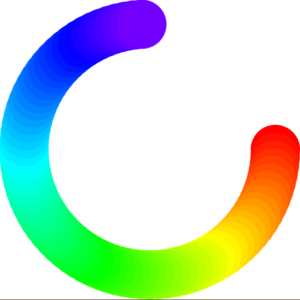Alongside, I plan to allow users to adjust the speed of the each newly added falling objects based on the values of an ultrasonic sensor. The idea is the closer the user is to the sensor/art, the more disturbance they cause to the artwork.

Additionally, I intend to incorporate sound and some additional output in form of LEDs. However, this is entirely dependent on the progress under time constraints amid finals.

In terms of the materials required, I plan to have a bit larger display than my 13inch laptop and some materials to aid with better technical design during the showcase.

```1x  >= 21'' screen
Multicolored LED strip/ LEDs (maybe)
1x  Knob
1x  Vertical Slider (could be DIY incase not available)
1x  Speaker (maybe)
1x  Ultrasonic Sensor

```

The hardest parts, I believe, in this project are the technical implementation and the efficient communication between the sensors and the code. For this, I have already started with coding the artwork and plan to slowly build up the features dependent on the analog sensors. As soon as possible, I plan to start working on building and assembling the user control system for the sensors for interaction.

## Final Project Proposal : Generative Art

Create a physically interactive system of your choice that relies on a multimedia computer for some sort of processing or data analysis. The Final should use BOTH Processing AND Arduino.

Idea:

I intend to design a generative art piece on processing using analog controls such as a potentiometer and distance sensor connected to Arduino. One such implementation could be to create a spiral where the potentiometer can control the direction and the distance sensor could control the design/ noise level/ thickness of the lines.

My inspiration are following example projects

The rotation of the squares is controlled by the potentiometer

The values from the pulse sensor are visualized in form of a sine wave generative art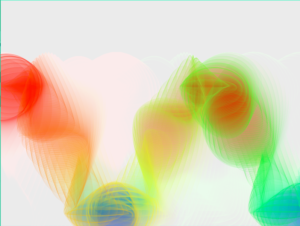Concerns:

At the moment, I am unsure if my project idea is good enough to be the final project for the course and if it will turn out to be as intended or not.

## Serial Communication Exercises

EXERCISE # 1

```//Arduino:

int left = 0;
int right = 0;

void setup() {
Serial.begin(9600);
Serial.println("0,0");
pinMode(2, OUTPUT);
pinMode(5, OUTPUT);
}

void loop() {
while (Serial.available()) {
right = Serial.parseInt();
left = Serial.parseInt();
digitalWrite(2, right);
digitalWrite(5, left);
delay(1);
delay(1);
Serial.print(sensor);
Serial.print(',');
Serial.println(sensor2);
}
}
}

/*Processing :

import processing.serial.*;
Serial myPort;
int xPos=0;
boolean onOff=false;
boolean onOff2=false;

void setup(){
size(960,720);
printArray(Serial.list());
String portname=Serial.list();
println(portname);
myPort = new Serial(this,portname,9600);
myPort.clear();
myPort.bufferUntil('\n');
}

void draw(){
background(255);
ellipse(xPos,height/2,30,30);
}

void serialEvent(Serial myPort){
s=trim(s);
if (s!=null){
println(s);
int values[]=int(split(s,','));
if (values.length==2){
xPos=(int)map(values,0,1023,0, width);
}
}
myPort.write(int(onOff)+","+int(onOff2)+"\n");
}
*/```

EXERCISE # 2

```//Arduino:

int brightness;

void setup() {
Serial.begin(9600);
Serial.println("0");
pinMode(3, OUTPUT);
}

void loop() {
while (Serial.available()) {
brightness = Serial.parseInt();
analogWrite(3, brightness);
}
}
}

/*
Processing:
import processing.serial.*;
Serial myPort;
int yPos=0;
float brightness;

void setup(){
size(960,720);
printArray(Serial.list());
String portname=Serial.list();
println(portname);
myPort = new Serial(this,portname,9600);
myPort.clear();
myPort.bufferUntil('\n');
}

void draw(){
background(255);
ellipse(width/2,mouseY,30,30);
brightness = map(mouseY,0,height,0,255);
myPort.write(int(brightness)+ "\n");
}

*/```

EXERCISE # 3

```//Arduino

int onoff;

void setup() {
Serial.begin(9600);
Serial.println("0");
pinMode(5, OUTPUT);
}

void loop() {
while (Serial.available()) {
onoff = Serial.parseInt();
digitalWrite(5, onoff);
delay(1);
Serial.println(sensor);
}
}
}

/*
Processing

import processing.serial.*;
Serial myPort;
PVector velocity;
PVector gravity;
PVector position;
PVector acceleration;
PVector wind;
float drag = 0.99;
float mass = 50;
float hDampening;
float windcontrolled;
boolean onoff = false;

void setup() {
size(640,360);
noFill();
position = new PVector(width/2, 0);
velocity = new PVector(0,0);
acceleration = new PVector(0,0);
gravity = new PVector(0, 0.5*mass);
wind = new PVector(0,0);
hDampening=map(mass,15,80,.98,.96);

//port communication
printArray(Serial.list());
String portname=Serial.list();
println(portname);
myPort = new Serial(this,portname,9600);
}

void draw() {
background(255);
if (!keyPressed){
wind.x=windcontrolled;
velocity.x*=hDampening;
}
applyForce(wind);
applyForce(gravity);
velocity.mult(drag);
acceleration.mult(0);
ellipse(position.x,position.y,mass,mass);
if (position.y > height-mass/2) {
velocity.y *= -0.9;  // A little dampening when hitting the bottom
position.y = height-mass/2;
}
if (position.y >= height-mass) {
onoff = true;
}
else{
onoff=false;
}

}

void serialEvent(Serial myPort){
s=trim(s);
if (s!=null){
int value = parseInt(s);
windcontrolled = map(value,0,1023,-1, 1);
}
myPort.write(int(onoff)+"\n");
}

void applyForce(PVector force){
// Newton's 2nd law: F = M * A
// or A = F / M
PVector f = PVector.div(force, mass);
}

void keyPressed(){
if (keyCode==LEFT){
wind.x=-1;
}
if (keyCode==RIGHT){
wind.x=1;
}
if (key==' '){
mass=random(15,80);
position.y=-mass;
velocity.mult(0);
}
}
*/```

EXERCISE # 3 VIDEO

## The Safe Room

Overview

This week, I designed a safe room using Arduino. The requirement was to create a circuit that will get information from at least one analog sensor and at least one digital sensor (switch) and use this information to control at least two LEDs, one in a digital fashion and the other in an analog fashion, in some creative way.

So, my creative idea involves a safe room that has a hidden secret controller. Every time someone enters the room, an intermediate warning is issued and the individual has 45 seconds to locate the hidden controller and adjust it to avoid triggering the security alarm. The location is highly confidential and only the top-tier managers know information. In case of an intruder, unless he is exceptionally smart enough to locate and adjust the controller, the person will be caught by this highly advanced security system.

Technical Implementation

One of the biggest challenges was to implement the idea on paper using the Arduino. After breaking into smaller steps, I figured it out. The digital switch was made using copper tape, one end on the board and one connected to the door. Once the door is open, it would send a HIGH to the connected digital input pin. Upon this, the always-on green light stops, and the yellow led starts glowing. Yellow led is controlled by the potentiometer (the controller). In the start it is set to a value of 1023 –  the bright yellow led and the individual has to switch off the led entirely using the potentiometer – to the value of 0. A timer is initiated as soon as the door is open and the individual has 45 seconds – 45000 milliseconds – to complete this. If the individual is successful, the green led turns back on. Otherwise, the red led is turned on digitally which signifies triggering the security alarm.

Here is the circuit:

Here’s the code:

```//Global variable
const int knob = A0;
const int red_led = 13;
const int yellow_led = 11;
const int green_led = 8;
const int input = 7;
int starttime;

void setup() {
//setting the modes
pinMode(red_led, OUTPUT);
pinMode(yellow_led, OUTPUT);
pinMode(green_led, OUTPUT);
pinMode(input, INPUT);

//start timer
starttime = millis();

Serial.begin(9600);

}

void loop() {

//when door is open
//turn off the green led
digitalWrite(green_led,LOW);
//turn the yellow led on
//control it anlogue way
int mappedvalue = map(knobValue,0,1023,255,0);
analogWrite(yellow_led,knobValue);

//keep count of the time elapsed since the door is open
Serial.print("millis elapsed : ");
Serial.println(millis()-starttime);
if((millis()-starttime) >= 45000){
//if not controlled within time, trigger the alarm
digitalWrite(red_led, HIGH);
}
//green switch back on successful completion
else{
digitalWrite(green_led, HIGH);
}
}
}
else{
//if door closed, keep yellow light off and green on
digitalWrite(yellow_led,LOW);
digitalWrite(green_led,HIGH);
Serial.println("You have 45 seconds to find and adjust the secret controller. Else alarm will go off!");
}
}
```

This is the video for when a known individual enters the room

This is in the case of an intruder

## Buzz Off: An Unusual Switch

In this production assignment, we had to create an unusual switch that does not require the use of hands and involves digital input and output for interaction. For this, I created a buzz game, where touching the course using the loop would close the switch. This, in turn, would stop the green LED and the red LED will start blinking.

This unusual game requires you to move the loop across the course using wrists. Sounds interesting but it is pretty difficult. I spent so much time simplifying and making the course yet interesting. I am yet to find someone that could complete this with the loop between wrists and without buzzing the circuit.

Here’s the complete circuit :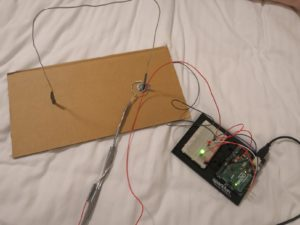Let’s play:

P.S Sorry for the bad video editing/ timing

The code is pretty simple and short. I have added a variable that keeps track of how many frames the switch has been closed –  a measure of how bad you are at this game.

```//global variables
int ledPingreen = 2;
int ledPinred = 4;
int input_ledPin = 7;
int touches = 0;
unsigned long timer = 0;
int timerAmount = 50;
bool flip = false;

void setup() {
// put your setup code here, to run once:
pinMode(input_ledPin, INPUT);
pinMode(ledPinred, OUTPUT);
pinMode(ledPingreen, OUTPUT);

//initiate timer
timer = millis();

//keep track of touches and monitor it
touches = 0;
Serial.begin(9600);

}

void loop() {
//monitoring touches
Serial.print("Number of touches until now : ");
Serial.println(touches);

//if the switch is closed
//increment number of touches
touches += 1;
if(millis()>= timer){
flip = !flip;
digitalWrite(ledPinred, flip);
timer = millis()+timerAmount;
}
//turn of green LED
digitalWrite(ledPingreen, LOW);
}

//if open
else{
//turn green LED on and red off
digitalWrite(ledPinred, LOW);
digitalWrite(ledPingreen, HIGH);
}
}```

## Processing Game : STRESS FREE NYUAD?

Overview

Finally, after all the ranting and hours of crying, the midterm game project is complete and it looks and works great, as intended, who am I kidding even. To summarize the game, the theme is around stress struggles as a student. To make it more personalized, the student is an NYUAD falcon. The game design includes free-falling stressful and stress-relieving activities that the player has to avoid and collect respectively while moving across the screen horizontally. It is a race between sanity and stress. The first total to reach 320 points wins. There are four types of free-falling activities that are worth a different number of points and allows the player certain boosted or dulled abilities. The game starts with the main page that allows the player to either start the game directly or navigate to the instructions screen. The game has three different difficulty levels that the player has to choose from. As the game concludes, a game over screen is displayed with results and the option to restart the game with a mouse press.

Production

This production process has been by far the most stressful, ironic right. With the code breaking down so many times, I started the initial game design from scratch with circles as the objects and incorporated the movement. See the initial sketch below.

Once the intended movement was achieved, building upon previous progress, I selected my images to use and incorporated them into the previous sketch. Finally, as shown below, the basic design of the game was complete.

The following steps included working more on the game outlook. Putting my artsy skills to use was real fun while coloring the free-falling objects png files. To have a better outlook, I used two images for the player, flipped left and right, which were chosen based on the movement of the player on the screen.

After completing this, the main landing screen, instructions screen, main gameplay screen, and game over screen were redesigned. For this, I used different background images, fonts, and text placements. Like most sketch screens, I wanted to have a faded image background during the main gameplay screen. However, with png moving down the screen, the background would distort, and also the increased loading time of the screen made the movement of objects weird and much less smooth. Therefore, after trying several different patterns and backgrounds, I just stick to a solid background. This came out looking pretty good design and allowing the moving objects’ color to pop on screen. Below are screenshots of the final designs.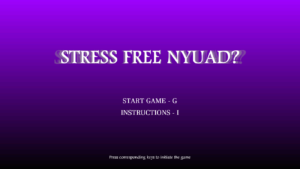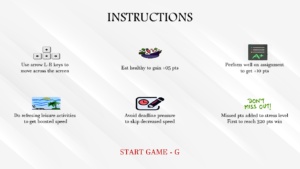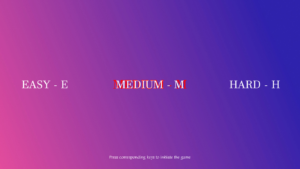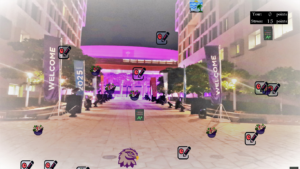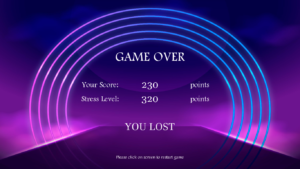Once all of the design was finalized, the last step was to include sound. There were challenges with different sounds playing at a time, ending up in weird noise. So, I finalized a single background sound looped until the game over screen and whenever a selection is made using mouse press or keypress, a notification sound is played. With this, I finalized my game design, and below is a demo

Code for the game is below (+500 lines) and the entire sketch can be downloaded from here

```import processing.sound.*;

//Global variables responsible for various states in game
//That includes: difficulty select, playing game, game over
Game start;
Player player;
String state = "MAIN";
String difficulty = "EASY";

//array to store the different points
Activity[] activityarray;

//all the images and fonts for design
PImage food;
PImage leisure;
PImage user;
PImage userr;
PImage bdrop;
PImage miss;
PImage bg;
PImage diff;
PImage inst;
PImage keys;
PImage back;
PFont f;
PFont g;

//global variables for location check and size
float speed = 1;
float previouspos = 0;

//soundfile
SoundFile audio;
SoundFile sel;

//setup the game

void setup(){

fullScreen();

//initializing game and player
start = new Game(state);
player = new Player();

back.resize(width,height);

audio = new SoundFile(this, "data/game.mp3");
audio.loop();
sel = new SoundFile(this, "data/select.mp3");

//font

//diffculty level
if(difficulty == "EASY"){
speed = 2;
}
if(difficulty == "MEDIUM"){
speed = 4;
}
if(difficulty == "HARD"){
speed = 8;
}

activityarray = new Activity;

//initializing the array of activity object
fillarray();

}

void draw(){
//image(back,0,0,width,height);
start.display();
}

//fill activity array
void fillarray(){
//choose random x values and drop activities
for(int i=0; i<activityarray.length; i++){
float x_pos = random(0,width-30);
while(x_pos == previouspos){
x_pos = random(0,width-30);
}
previouspos = x_pos;
float type = random(1,50);
String activity_ability = "FOOD";

//randomly choosing  ability based on random number
if(type < 5){
activity_ability = "LEISURE";
}
else if(type > 45){
}
if(type > 10 && type <=25){
}

//create new activity object
activityarray[i] = new Activity(x_pos,random(-10000,0),speed,activity_ability);
}
}

//game class
class Game{
String mode;
int score = 0;
int lostpoints = 0;
float speed = 0;

Game(String state){
mode = state;
}

void display(){
textAlign(CENTER,CENTER);

//check for game over
if(lostpoints >= 320 || score >= 320){
mode = "OVER";
audio.stop();
}

if(mode=="MAIN"){

background(255);
for(int y=0;y<height;y++){
for(int x=0;x<width; x++){
int index = x+y*width;
pixels[index] = color(map(y,0,height,155,0),0,map(y,0,height,255,0),50);
}
}
updatePixels();

//displaying text
textFont(f,random(90,97));
fill(150,120);
fill(255);
textFont(f,90);
textFont(g,30);
fill(255);
text("START GAME - G",width/2,2*height/3 - height/14);
text("INSTRUCTIONS - I",width/2,2*height/3);
textFont(f,20);
text("Press corresponding keys to initiate the game",width/2,height-height/14);
}

if(mode=="INSTRUCTIONS"){
fill(0);
image(inst,0,0,width,height);
textFont(f,60);
text("INSTRUCTIONS",width/2,height/10);

//display images and text
image(keys,width/4-width/7,height/2.5 - height/8,130,60);
image(food,width/2-130/2,height/2.5 - height/8,130,60);
image(leisure,width/4-width/7,1.75*height/2.5 - height/8,130,60);
image(miss,3*width/4+width/16,1.75*height/2.5 - height/8,130,60);
textFont(g,22);
text("Use arrow L-R keys to\nmove across the screen",width/4-width/10,height/2.5);
text("Eat healthy to gain +05 pts",width/2,height/2.5);
text("Perform well on assignment\n to get +10 pts ",3*width/4+width/10,height/2.5);
text("Do refresing leisure activities\n to get boosted speed",width/4-width/10,1.75*height/2.5);
text("Avoid deadline pressure\nto skip decreased speed",width/2,1.75*height/2.5);
text("Missed pts added to stress level\nFirst to reach 320 pts win",3*width/4+width/10,1.75*height/2.5);
textFont(g,random(32,34));
fill(random(255),0,0);
text("START GAME - G",width/2,9*height/10);
}

//displaying the difficulty selection screen
if(mode=="DIFFICULTY"){
image(diff,0,0,width,height);
//turn the text red and create shadow effect when mouse is hovered
//over the level selection part
if(mouseX>0 && mouseX<width/3){
fill(255,0,0);
textFont(g,54);
text("EASY - E",width/4-width/10,height/2);
}
else if(mouseX>width/3 && mouseX<2*width/3){
fill(255,0,0);
textFont(g,54);
text("MEDIUM - M",width/2,height/2);
}
else if(mouseX>2*width/3 && mouseX<width){
fill(255,0,0);
textFont(g,54);
text("HARD - H",3*width/4+width/10,height/2);
}
fill(255);
textFont(g,50);
text("EASY - E",width/4-width/10,height/2);
text("MEDIUM - M",width/2,height/2);
text("HARD - H",3*width/4+width/10,height/2);
textFont(f,20);
text("Press corresponding keys to initiate the game",width/2,height-height/14);

}

//game over screen
if(mode=="OVER"){
//display the background image
fill(255);
image(bg,0,0,width,height);

//display the text
textFont(f,60);
text("GAME OVER",width/2, height/3);
textFont(f,35);
text("Your Score:",width/2 - width/6, height/3 +height/6);
text("Stress Level:",width/2 - width/6, height/3 + height/4);
textFont(g,45);
text(score,width/2, height/3 +height/6);
text(lostpoints,width/2, height/3 + height/4);
textFont(f,35);
text("points",width/2 + width/6, height/3 +height/6);
text("points",width/2 +width/6, height/3 + height/4);

//display result string based on scores
textFont(g,45);
if(score>=lostpoints){
if(score==lostpoints){
text("IT'S A TIE", width/2, 2.25*height/3);
}
else{
text("YOU WON", width/2, 2.25*height/3);
}
}
else{
text("YOU LOST", width/2, 2.25*height/3);
}
textFont(f,20);
text("Please click on screen to restart game",width/2,height-height/14);
}

//main game screen
if(mode=="PLAY"){

//background color
background(back);
fill(0);

//score board
rect(width-width/6,height/14.5,width/8,height/13.5);
fill(255);
textFont(g,18);
text("Your:", width-width/7,height/12);
text("Stress:", width-width/7,height/8);
textFont(f,random(24,26));
fill(random(200,255));
text(score,width-width/10,height/12);
text(lostpoints,width-width/10,height/8);
fill(255);
textFont(g,18);
text("points", width-width/16.5,height/12);
text("points", width-width/16.5,height/8);

//main display player
player.display();

//display activities
for(int i =0; i< activityarray.length;i++)
{
activityarray[i].display();
if(activityarray[i].yloc > height){
lostpoints += activityarray[i].point;
activityarray[i].point = 0;
}

//resize the image upon collision to have effect
//of collecting the activity
if(activityarray[i].collisions() == true){
score += activityarray[i].point;
activityarray[i].awidth = 0;
activityarray[i].aheight = 0;
activityarray[i].point =0;
}
}
}
}
}

//player class
class Player{
float pwidth;
float pheight;
float xPos;
float yPos;
boolean left;
boolean right;
float speed;
float fast_time;
float slow_time;

Player(){
pwidth= 100;
pheight = 100;
xPos = width/2 - pwidth;
yPos = height - pheight;
left = false;
right = false;
speed = 7;
fast_time = 0;
slow_time = 0;
}

void display(){

//tracking the time when boosted speed
if(speed == 12){
fast_time += 1;
//last 100 frames
if(fast_time == 100){
fast_time = 0;
speed = 7;
}
}

//tracking the time when slowed speed
if(speed == 1){
slow_time += 1;
//last 100 frames
if(slow_time == 100){
slow_time = 0;
speed = 7;
}
}

//update the position on screen
update();

//draw the player
if(left==true){
image(user,xPos,yPos,pwidth,pheight);
}
else if(right==true){
image(userr,xPos,yPos,pwidth,pheight);
}
else{
image(userr,xPos,yPos,pwidth,pheight);
}
}

//update the position of the player
void update(){
if(left==true && xPos >=0){
xPos -= speed;
}
if(right==true && xPos <= width-pwidth){
xPos += speed;
}
}
}

//Class of falling activities/ points
class Activity{
float awidth = 60;
float aheight = 60;
//coordinates
float yloc;
float xloc;
float speed;
String ability;
//standard point
int point = 5;
//image
PImage activityimg;

Activity(float xpos, float y,float s, String a){
xloc = xpos;
speed= s;
yloc = y;
ability = a;

//updating point values and image based on type
point = 10;
}
else if(ability == "LEISURE"){
activityimg = leisure;
}
point = 0;
}
else{
point =5;
activityimg = food;
}
}

//display the activity object
void display(){
update();
image(activityimg,xloc,yloc,awidth,aheight);
}

//update the locations
void update(){
//move down
yloc += speed;
}

//check for collisions
boolean collisions(){
if((player.xPos + player.pwidth >= xloc) && (player.xPos <= xloc + awidth)){
if((yloc + aheight >= player.yPos) && (yloc <= player.pheight + player.yPos)){

//check if it collides with special activity and update speed accordingly
if(ability == "LEISURE"){
player.speed = 12;
}
player.speed = 1;
}
return true;
}
}
return false;
}
}

//keep track of key presses on screen
void keyPressed(){
if(start.mode == "MAIN"){
if(keyCode == 73){      //73 = 'i'
sel.play();
start.mode = "INSTRUCTIONS";
}
if(keyCode == 71){        //71 = 'g'
sel.play();
start.mode = "DIFFICULTY";
}
}

if(start.mode == "INSTRUCTIONS"){
if(keyCode == 71){        //71 = 'g'
sel.play();
start.mode = "DIFFICULTY";
}
}

if(start.mode == "DIFFICULTY"){
if(keyCode == 69){        //71 = 'e'
sel.play();
difficulty = "EASY";
start.mode = "PLAY";
}
if(keyCode == 77){        //71 = 'm'
sel.play();
difficulty = "MEDIUM";
start.mode = "PLAY";
}
if(keyCode == 72){        //71 = 'h'
sel.play();
difficulty = "HARD";
start.mode = "PLAY";
}
}

//move until key pressed
if(start.mode=="PLAY"){
if(keyCode == RIGHT){
player.right = true;
}
if(keyCode == LEFT){
player.left = true;
}
}

}

//stop motion when key is released
void keyReleased(){
if(start.mode == "PLAY"){
if(keyCode == RIGHT){
player.right = false;
}
if(keyCode == LEFT){
player.left = false;
}
}
}

//replay the game
void mouseClicked(){
if(start.mode=="OVER"){
sel.play();
player.left = false;
player.right = false;
fillarray();
background(0);
start = new Game("MAIN");
audio.play();
}
}```

## Midterm Project Progress

As part of the midterm project, I am designing a game around the theme of coping with stress as an NYUAD student. It was really difficult for me to settle on one single idea as I found myself trying several different things for hours and then ultimately deciding on this idea finally a night before the submission is due.  The intended game design is to collect the falling activities (that increase or help cope with stress levels). Each activity is associated with a certain number of points and special abilities such as boosted/ slowed down the speed of the player. The player moves across the screen horizontally and tries to catch points. All missed points are added to the stress level and if the stress level reaches 320, the player loses. If the player collects 320 points first, he/she wins. The user is allowed the option to restart the game also.

Production

To start with the implementation, I sketched out some layouts of my instruction screen and main page.

I then created a simplistic version of these pages to later come back and design them. The whole navigation across the game is based on keypresses and mouse presses. Specific keys are set to allow the selection in the game and proceed forward with the setting. Below are the snapshots:

Here is the code worked on until now

```//Global variables responsible for various states in game
//That includes: difficulty select, playing game, game over
Game start;
Player player;
String state = "MAIN";
String difficulty = "EASY";
Activity[] activityarray;

//setup the game
void setup(){
size(600,450);
start = new Game(state, difficulty);
activityarray = new Activity;
//choose random x values and drop activities
for(int i=0; i<activityarray.length; i++){
float x_pos = random(0,width-50);
float type = random(1,50);
String activity_ability = "FOOD";
//randomly choosing ability
if(type < 5){
activity_ability = "LEISURE";
}
else if(type > 45){
}
if(type > 10 && type <=25){
}
//create new activity object
activityarray[i] = Activity(x_pos,start.speed,activity_ability);
}
}

//game class
class Game{
String mode;
String level;
int score = 0;
int lostpoints = 0;
float speed = 0;
float drop_speed = 30;
float frames = 0;

Game(String state, String difficulty){
mode = state;
level = difficulty;
}

void display(){
textAlign(CENTER,CENTER);

//check for game over
if(lostpoints >= 320 || score >= 320){
mode = "OVER";
}

if(mode=="MAIN"){
for(int y=0;y<height;y++){
for(int x=0;x<width; x++){
int index = x+y*width;
pixels[index] = color(map(y,0,height,155,0),0,map(y,0,height,255,0),50);
}
}
updatePixels();
textSize(50);
text("//GAME NAME",width/2,height/3);
textSize(18);
text("START GAME (G)",width/2,2*height/3 - height/14);
text("INSTRUCTIONS (I)",width/2,2*height/3);
textSize(14);
text("Press corresponding keys to initiate the game",width/2,height-height/14);
}

if(mode=="INSTRUCTIONS"){
background(120);
textSize(30);
text("//INSTRUCTIONS",width/2,height/10);
textSize(15);
text("//Use arrow keys to move across the screen",width/2,2.5*height/10);
text("//Eat healthy to gain +10 pts",width/2,3.5*height/10);
text("//Perform well on assignment to get +20 pts ",width/2,4.5*height/10);
text("//Do refresing leisure activities to get boosted speed",width/2,5.5*height/10);
text("//Missed pts are added to stress level. First to reach 320 pts win",width/2,7.5*height/10);
textSize(22);
text("//START GAME (G)",width/2,9*height/10);
}

if(mode=="DIFFICULTY"){
background(120);
textSize(30);
text("//DIFFICULTY LEVEL",width/2,height/10);
textSize(20);
text("//EASY (E)",width/2,3.5*height/10);
text("//MEDIUM (M)",width/2,4.5*height/10);
text("//HARD (H)",width/2,5.5*height/10);
}

//game over screen
if(mode=="OVER"){
background(150);
textSize(40);
text("GAME OVER",width/2, height/5);
textSize(25);
text("Your Score:",width/2 - width/6, height/3 +height/15);
text("Stress Level:",width/2 -width/6, height/3 + height/7);
text(score,width/2, height/3 +height/15);
text(lostpoints,width/2, height/3 + height/7);
text("points",width/2 + width/6, height/3 +height/15);
text("points",width/2 +width/6, height/3 + height/7);
if(score>=lostpoints){
if(score==lostpoints){
text("IT'S A TIE\nWanna try again?", width/2, 2*height/3);
}
else{
text("YOU WON", width/2, 2*height/3);
}
}
else{
text("YOU LOST\nWanna try again?", width/2, 2*height/3);
}
textSize(15);
text("//Please click on screen to restart game",width/2,9*height/10);
}

//main game screen
if(mode=="PLAY"){
background(255);

}
}
}

//player class
class Player{
float pwidth= 100;
float pheight = 100;
float base = 600;
float xPos = width/2 - pwidth/2;
float yPos = height - (height - base) - pheight;
boolean left = false;
boolean right = false;
float speed = 5;
float fast_time = 0;
float slow_time = 0;

Player(){}

void display(){

//tracking the time when boosted speed
if(speed == 10){
fast_time += 1;
//last 100 frames
if(fast_time == 100){
fast_time = 0;
speed = 5;
}
}

//tracking the time when slowed speed
if(speed == 1){
fast_time += 1;
//last 100 frames
if(fast_time == 100){
fast_time = 0;
speed = 5;
}
}

//update the position on screen
update();

//change color based on ability
if(speed == 1){
tint(214,87,77);
}
else if(speed == 10){
tint(237,211,81);
}

//draw the player
fill(50,0,50);
ellipse(xPos,yPos,pwidth,pheight);

//stop tint
noTint();
}

//update the position of the player
void update(){
if(left==true && xPos >=0){
xPos -= speed;
}
if(right==true && xPos <= width - pwidth){
xPos += speed;
}
}

}

//Class of falling activities/ points
class Activity{

//resizing required
float awidth = 50;
float aheight = 40;
//coordinates
float yloc = -aheight;
float xloc;
float speed;
String ability;
//standard point
int point = 10;
//image
PImage activityimg;

Activity(float xpos, float s, String a){
xloc = xpos;
speed = s;
ability = a;

//updating point values
point = 20;
}
else if(ability == "LEISURE"){
}
point = 0;
}
else{
}
}
//display the images
void display(){
image(activityimg,xloc,yloc,awidth,aheight);
}

//update the locations
void update(){
//move down
yloc += speed;
}

//check for collisions
boolean collisions(){
if((player.xPos + player.pwidth >= xloc) && (player.xPos <= xloc + awidth)){
if((yloc + aheight >= player.yPos) && (yloc <= player.pheight + player.yPos)){

//check if it collides with special activity
if(ability == "LEISURE"){
player.speed = 10;
}
player.speed = 1;
}
return true;
}
}
return false;
}
}

void draw(){
start.display();
}

//keep track of key presses on screen
void keyPressed(){
if(start.mode == "MAIN"){
if(keyCode == 73){        //73 = 'i'
start.mode = "INSTRUCTIONS";
}
if(keyCode == 71){        //71 = 'g'
start.mode = "DIFFICULTY";
}
}

if(start.mode == "INSTRUCTIONS"){
if(keyCode == 71){        //71 = 'g'
start.mode = "DIFFICULTY";
}
}

if(start.mode == "DIFFICULTY"){
if(keyCode == 69){        //71 = 'e'
difficulty = "EASY";
start.speed = 3;
start.mode = "PLAY";
}
if(keyCode == 77){        //71 = 'm'
difficulty = "MEDIUM";
start.speed = 6;
start.mode = "PLAY";
}
if(keyCode == 72){        //71 = 'h'
difficulty = "HARD";
start.speed = 10;
start.mode = "PLAY";
}
}

//move until key pressed
if(start.mode=="PLAY"){
if(keyCode == RIGHT){
player.right = true;
}
if(keyCode == LEFT){
player.left = true;
}
}

}

//stop motion when key is released
void keyReleased(){
if(start.mode == "PLAY"){
if(keyCode == RIGHT){
player.right = false;
}
if(keyCode == LEFT){
player.left = false;
}
}
}

//replay the game
void mouseClicked(){
if(start.mode=="OVER"){

start.mode = "MAIN";
noTint();
start = new Game("MAIN","EASY");
}
}```

Challenges

Aside from taking so long to decide on one single project, I have encountered several issues. First and foremost, my whole game can not be executed once all the classes have been defined and all the code has been linked together. I have done my best to make as much progress I could on the overall game, but I am hoping with a few more hours of work, I’ll be able to finish off linking my code and making it work.

Firstly, I need to finish off the basic code and allow for the basic functioning of the game to be complete. Then, I need to work on the design and aesthetics of the screen and overall game. Lastly, I need to resize my images and add sound to the game.

## Data Visualization Assignment

Overview

In this technologically and scientifically advanced era, there is no shortage of data of any sort, for most cases. For this production assignment, I used a online available database of the world’s cities and towns. It has been built from the ground up using authoritative sources such as the NGIA, US Geological Survey, US Census Bureau, and NASA. (https://simplemaps.com/data/world-cities)

Using the information mentioned in the database about 41001 cities, I created a world map on Processing. It creates two maps: based on total population size and one solely based on location. Initially, when it loads, the user might be able to only understand it as a random placement of randomly sized circles that are a bit wobbly but when the mouse pressed the other map that marks the location of all 41001 cities, an evident world map forms.

Here’s a quick video overview

Production

Initially, I had no clue what sort of data visualization project I want to create. While randomly browsing large datasets available online, I came across this database and thought of using it to create the world map. I used the latitude and longitude values of a city to manipulate their position on the canvas in terms of y and x respectively.

For a long while, I stared at my blank screen wondering what went wrong when only a few locations would appear. It took me a lot of trial and error and maths and geography of course to understand the mapping of longitude and latitude values to cartesian values. After identifying a pattern, I formed pretty simple mathematical equations for this, and there you go, the world map appears.

To add more clarity and interactivity, I allowed the user to hold the mouse and see the whole world map. For this implementation, I created two arrays for city circle objects. Each city circle object has a Pvector to store its x and y values, its randomly decided color and transparency, and its size. The only difference in both arrays is the size of the circles. The first array that displays on canvas has size-dependent on population size (total population / 700000) and the other one has a fixed size of 1px to allow for visibility of all. The wobbly movement is achieved by using random() on the size of the circle. Additionally, the user can see both maps together by pressing the SHIFT key.

Heres the code

```//global variables
CityCircle[] cities;
CityCircle[] all;
Table world_cities;
boolean together = false;

void setup(){
size(720,360);
frameRate(10);
noStroke();
}

void draw(){
background(255);
for(int i=0; i<cities.length; i++){
cities[i].display();
}

//appear entire world map on mouse press
if (mousePressed){
background(255);
for(int i=0; i<cities.length; i++){
all[i].display();
}
}

//appear together
if (together==true){
for(int i=0; i<cities.length; i++){
all[i].display();
}
}

if (keyPressed && keyCode==SHIFT){
together = true;
}

}

//initializing the arrays
cities = new CityCircle[world_cities.getRowCount()];
all = new CityCircle[world_cities.getRowCount()];

//intialize values of each city object
for (int i = 0; i < world_cities.getRowCount(); i++) {
TableRow row = world_cities.getRow(i);

//Acessing the fields via their column name
String n = row.getString("city");
float y = row.getFloat("lat");
float x = row.getFloat("lng");
float s = row.getFloat("population");

// Make a Circle object out of the data read
PVector p = new PVector(360 + x*2,180-y*2);
//colour for first array
color c = color(random(255),150,random(255),100);
cities[i] = new CityCircle(p,n,s/500000,c);
//color for second array
color c2= color(random(255),100,random(255),200);
all[i] = new CityCircle(p,n,1,c2);
}

}

//Object class
class CityCircle{
PVector position;
String name;
float size;
color colour;

CityCircle(PVector p, String n, float s, color c){
position = p;
name = n;
size = s;
colour = c;
}

void display(){
fill(colour);
circle(position.x,position.y,random(size-.75,size+.75));
}

}```